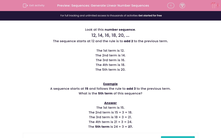# Sequences: Generate Linear Number Sequences

In this worksheet, students generate linear number sequences from the given instructions and formulae.Key stage:  KS 2

Curriculum topic:   Maths and Numerical Reasoning

Curriculum subtopic:   Sequences

Difficulty level:#### Worksheet Overview

Look at this number sequence.

12, 14, 16, 18, 20, ...

The sequence starts at 12 and the rule is to add 2 to the previous term.

The 1st term is 12.

The 2nd term is 14.

The 3rd term is 16.

The 4th term is 18.

The 5th term is 20.

Example

A sequence starts at 15 and follows the rule to add 3 to the previous term.

What is the 5th term of this sequence?

The 1st term is 15.

The 2nd term is 15 + 3 = 18.

The 3rd term is 18 + 3 = 21.

The 4th term is 21 + 3 = 24.

The 5th term is 24 + 3 = 27.

### What is EdPlace?

We're your National Curriculum aligned online education content provider helping each child succeed in English, maths and science from year 1 to GCSE. With an EdPlace account you’ll be able to track and measure progress, helping each child achieve their best. We build confidence and attainment by personalising each child’s learning at a level that suits them.

Get started